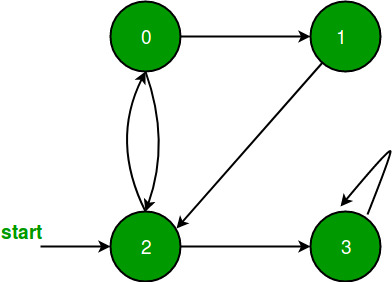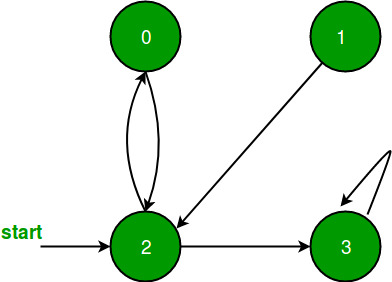Related Articles
BFS for Disconnected Graph
• Difficulty Level : Easy
• Last Updated : 17 Feb, 2020

In previous post, BFS only with a particular vertex is performed i.e. it is assumed that all vertices are reachable from the starting vertex. But in the case of disconnected graph or any vertex that is unreachable from all vertex, the previous implementation will not give the desired output, so in this post, a modification is done in BFS.All vertices are reachable. So, for above graph simple BFS will work.As in above graph a vertex 1 is unreachable from all vertex, so simple BFS wouldn’t work for it.

```Just to modify BFS, perform simple BFS from each
unvisited vertex of given graph.
```

## C++

 `// C++ implementation of modified BFS``#include``using` `namespace` `std;`` ` `// A utility function to add an edge in an``// undirected graph.``void` `addEdge(vector<``int``> adj[], ``int` `u, ``int` `v)``{``    ``adj[u].push_back(v);``}`` ` `// A utility function to do BFS of graph``// from a given vertex u.``void` `BFSUtil(``int` `u, vector<``int``> adj[],``            ``vector<``bool``> &visited)``{`` ` `    ``// Create a queue for BFS``    ``list<``int``> q;``  ` `    ``// Mark the current node as visited and enqueue it``    ``visited[u] = ``true``;``    ``q.push_back(u);``  ` `    ``// 'i' will be used to get all adjacent vertices 4``    ``// of a vertex list::iterator i;``  ` `    ``while``(!q.empty())``    ``{``        ``// Dequeue a vertex from queue and print it``        ``u = q.front();``        ``cout << u << ``" "``;``        ``q.pop_front();``  ` `        ``// Get all adjacent vertices of the dequeued``        ``// vertex s. If an adjacent has not been visited, ``        ``// then mark it visited and enqueue it``        ``for` `(``int` `i = 0; i != adj[u].size(); ++i)``        ``{``            ``if` `(!visited[adj[u][i]])``            ``{``                ``visited[adj[u][i]] = ``true``;``                ``q.push_back(adj[u][i]);``            ``}``        ``}``    ``}``}`` ` `// This function does BFSUtil() for all ``// unvisited vertices.``void` `BFS(vector<``int``> adj[], ``int` `V)``{``    ``vector<``bool``> visited(V, ``false``);``    ``for` `(``int` `u=0; u adj[V];`` ` `    ``addEdge(adj, 0, 4);``    ``addEdge(adj, 1, 2);``    ``addEdge(adj, 1, 3);``    ``addEdge(adj, 1, 4);``    ``addEdge(adj, 2, 3);``    ``addEdge(adj, 3, 4);``    ``BFS(adj, V);``    ``return` `0;``}`

## Java

 `// Java implementation of modified BFS ``import` `java.util.*;``public` `class` `graph ``{``    ``//Implementing graph using HashMap``    ``static` `HashMap> graph=``new` `HashMap<>();`` ` `    ``//utility function to add edge in an undirected graph``public` `static` `void` `addEdge(``int` `a,``int` `b)``{``    ``if``(graph.containsKey(a))``    ``{``        ``LinkedList l=graph.get(a);``        ``l.add(b);``        ``graph.put(a,l);``    ``}``    ``else``    ``{``        ``LinkedList l=``new` `LinkedList<>();``        ``l.add(b);``        ``graph.put(a,l);``    ``}``}`` ` `//Helper function for BFS ``public` `static` `void` `bfshelp(``int` `s,ArrayList visited)``{``    ``// Create a queue for BFS ``    ``LinkedList q=``new` `LinkedList<>();``     ` `    ``// Mark the current node as visited and enqueue it ``    ``q.add(s);``    ``visited.set(s,``true``);``     ` `    ``while``(!q.isEmpty())``    ``{``        ``// Dequeue a vertex from queue and print it ``        ``int` `f=q.poll();``        ``System.out.print(f+``" "``);``         ` `        ``//Check whether the current node is ``                ``//connected to any other node or not``        ``if``(graph.containsKey(f))``        ``{``        ``Iterator i=graph.get(f).listIterator();``         ` `        ``// Get all adjacent vertices of the dequeued ``        ``// vertex f. If an adjacent has not been visited,  ``        ``// then mark it visited and enqueue it ``         ` `            ``while``(i.hasNext())``            ``{``                ``int` `n=i.next();``                ``if``(!visited.get(n))``                ``{``                ``visited.set(n,``true``);``                ``q.add(n);``                ``}``            ``}``        ``}``    ``}``     ` `}`` ` `//BFS function to check each node``public` `static` `void` `bfs(``int` `vertex)``{``    ``ArrayList visited=``new` `ArrayList();``    ``//Marking each node as unvisited``    ``for``(``int` `i=``0``;i

## Python3

 `# Python3 implementation of modified BFS ``import` `queue`` ` `# A utility function to add an edge ``# in an undirected graph. ``def` `addEdge(adj, u, v):``    ``adj[u].append(v)`` ` `# A utility function to do BFS of ``# graph from a given vertex u. ``def` `BFSUtil(u, adj, visited):`` ` `    ``# Create a queue for BFS ``    ``q ``=` `queue.Queue()``     ` `    ``# Mark the current node as visited``    ``# and enqueue it ``    ``visited[u] ``=` `True``    ``q.put(u) ``     ` `    ``# 'i' will be used to get all adjacent ``    ``# vertices 4 of a vertex list::iterator i ``     ` `    ``while``(``not` `q.empty()):``         ` `        ``# Dequeue a vertex from queue ``        ``# and print it ``        ``u ``=` `q.queue[``0``] ``        ``print``(u, end ``=` `" "``) ``        ``q.get() ``     ` `        ``# Get all adjacent vertices of the ``        ``# dequeued vertex s. If an adjacent ``        ``# has not been visited, then mark ``        ``# it visited and enqueue it ``        ``i ``=` `0``        ``while` `i !``=` `len``(adj[u]):``            ``if` `(``not` `visited[adj[u][i]]):``                    ``visited[adj[u][i]] ``=` `True``                    ``q.put(adj[u][i])``            ``i ``+``=` `1`` ` `# This function does BFSUtil() for all ``# unvisited vertices. ``def` `BFS(adj, V):``    ``visited ``=` `[``False``] ``*` `V ``    ``for` `u ``in` `range``(V):``        ``if` `(visited[u] ``=``=` `False``): ``            ``BFSUtil(u, adj, visited)`` ` `# Driver code ``if` `__name__ ``=``=` `'__main__'``:`` ` `    ``V ``=` `5``    ``adj ``=` `[[] ``for` `i ``in` `range``(V)] `` ` `    ``addEdge(adj, ``0``, ``4``) ``    ``addEdge(adj, ``1``, ``2``) ``    ``addEdge(adj, ``1``, ``3``) ``    ``addEdge(adj, ``1``, ``4``) ``    ``addEdge(adj, ``2``, ``3``) ``    ``addEdge(adj, ``3``, ``4``) ``    ``BFS(adj, V)`` ` `# This code is contributed by PranchalK`

## C#

 `// C# implementation of modified BFS ``using` `System;``using` `System.Collections.Generic;`` ` `class` `Graph ``{ ``    ``//Implementing graph using Dictionary ``    ``static` `Dictionary<``int``,List<``int``>> graph = ``            ``new` `Dictionary<``int``,List<``int``>>(); `` ` `//utility function to add edge in an undirected graph ``public` `static` `void` `addEdge(``int` `a, ``int` `b) ``{ ``    ``if``(graph.ContainsKey(a)) ``    ``{ ``        ``List<``int``> l = graph[a]; ``        ``l.Add(b); ``        ``if``(graph.ContainsKey(a))``            ``graph[a] = l;``        ``else``            ``graph.Add(a,l); ``    ``} ``    ``else``    ``{ ``        ``List<``int``> l = ``new` `List<``int``>(); ``        ``l.Add(b); ``        ``graph.Add(a, l); ``    ``} ``} `` ` `// Helper function for BFS ``public` `static` `void` `bfshelp(``int` `s, List visited) ``{ ``    ``// Create a queue for BFS ``    ``List<``int``> q = ``new` `List<``int``>(); ``     ` `    ``// Mark the current node as visited and enqueue it ``    ``q.Add(s); ``    ``visited.RemoveAt(s);``    ``visited.Insert(s,``true``); ``     ` `    ``while``(q.Count != 0) ``    ``{ ``        ``// Dequeue a vertex from queue and print it ``        ``int` `f = q; ``        ``q.RemoveAt(0);``        ``Console.Write(f + ``" "``); ``         ` `        ``//Check whether the current node is ``        ``//connected to any other node or not ``        ``if``(graph.ContainsKey(f)) ``        ``{ ``         ` `        ``// Get all adjacent vertices of the dequeued ``        ``// vertex f. If an adjacent has not been visited, ``        ``// then mark it visited and enqueue it ``         ` `            ``foreach``(``int` `iN ``in` `graph[f]) ``            ``{ ``                ``int` `n = iN; ``                ``if``(!visited[n]) ``                ``{ ``                    ``visited.RemoveAt(n);``                    ``visited.Insert(n, ``true``); ``                    ``q.Add(n); ``                ``} ``            ``} ``        ``} ``    ``} ``     ` `} `` ` `// BFS function to check each node ``public` `static` `void` `bfs(``int` `vertex) ``{ ``    ``List visited = ``new` `List(); ``     ` `    ``// Marking each node as unvisited ``    ``for``(``int` `i = 0; i < vertex; i++) ``    ``{ ``        ``visited.Insert(i, ``false``); ``    ``} ``    ``for``(``int` `i = 0; i < vertex; i++) ``    ``{ ``        ``// Checking whether the node is visited or not ``        ``if``(!visited[i]) ``        ``{ ``            ``bfshelp(i, visited); ``        ``} ``    ``} ``} `` ` `// Driver Code ``public` `static` `void` `Main(String[] args) ``{ ``    ``int` `v = 5; ``    ``addEdge(0, 4); ``    ``addEdge(1, 2); ``    ``addEdge(1, 3); ``    ``addEdge(1, 4); ``    ``addEdge(2, 3); ``    ``addEdge(3, 4); ``    ``bfs(v); ``} ``} `` ` `// This code is contributed by Rajput-Ji`

Output:

```0 4 1 2 3
```

This article is contributed by Sahil Chhabra (akku). If you like GeeksforGeeks and would like to contribute, you can also write an article using contribute.geeksforgeeks.org or mail your article to contribute@geeksforgeeks.org. See your article appearing on the GeeksforGeeks main page and help other Geeks.